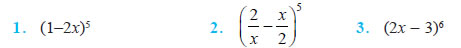# Binomial Theorem Class 11 NCERT Solutions

NCERT Solutions Class 11 Mathematics Chapter 8 Binomial Theorem Download In Pdf

## In this pdf file you can see answers of following Questions

### EXERCISE 8.1

Expand each of the expressions in Exercises 1 to 5. Using binomial theorem, evaluate each of the following:Question 6. (96)3

Question 7. (102)5

Question 8. (101)4

Question 9. (99)5

Question 10. Using Binomial Theorem, indicate which number is larger (1.1)10000 or 1000.

Question 11. Find (a + b)4 – (a – b)4. Hence, evaluate ( 3 + 2)4– ( 3 – 2)4 .

Question 12. Find (x + 1)6 + (x – 1)6. Hence or otherwise evaluate ( 2 + 1)6 + ( 2 – 1)6.

Question 13. Show that 9n+1 – 8n – 9 is divisible by 64, whenever n is a positive integer.

### EXERCISE 8.2

Find the coefficient of

Question 1. x5 in (x + 3)8 2. a5b7 in (a – 2b)1

Question 2. Write the general term in the expansion of

Question 3. (x2 – y)6

Question 4. (x2 – yx)12, x ≠ 0.

Question 5. Find the 4th term in the expansion of (x – 2y)12.

Question 6. Find the 13th term in the expansion of 18 9 1 3 x x , x ≠ 0. Find the middle terms in the expansions of

Question 7. 3 7 6 3 − x

Question 8. 10 9 3 x + y

Question 9. In the expansion of (1 + a)m+n, prove that coefficients of am and an are equal.

Question 10. The coefficients of the (r – 1)th, rth and (r + 1)th terms in the expansion of (x + 1)n are in the ratio 1 : 3 : 5. Find n and r.

Question 11. Prove that the coefficient of xn in the expansion of (1 + x)2n is twice the coefficient of xn in the expansion of (1 + x)2n – 1.

Question 12. Find a positive value of m for which the coefficient of x2 in the expansion (1 + x)m is 6.

### NCERT Books Free Pdf Download for Class 5, 6, 7, 8, 9, 10 , 11, 12 Hindi and English Medium

 Mathematics Biology Psychology Chemistry English Economics Sociology Hindi Business Studies Geography Science Political Science Statistics Physics Accountancy